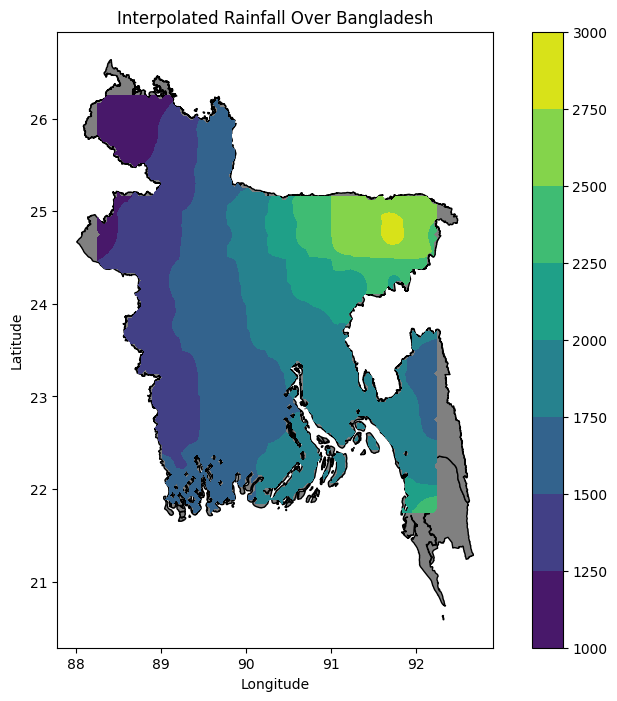## Spatial Interpolation of station data¶

#### Authors:¶

###### Noshin Sharmili (nvs5791@psu.edu; noshinsharmili0@gmail.com)¶
In [ ]:
# Not all packages run below required here. Some of them here for test purposes.

In [ ]:
import numpy as np
import pandas as pd
import geopandas as gpd
import matplotlib.pyplot as plt
import matplotlib.patches as patches
import matplotlib.ticker as mticker
import cartopy.crs as ccrs
from scipy.interpolate import griddata
from scipy.spatial import distance_matrix
from scipy.interpolate import LinearNDInterpolator
from shapely.geometry import Point
from pykrige.ok import OrdinaryKriging
from rasterio.transform import from_origin
from sklearn.preprocessing import PolynomialFeatures
from sklearn.linear_model import LinearRegression
from sklearn.pipeline import make_pipeline
from scipy.interpolate import Rbf
from sklearn.metrics.pairwise import haversine_distances
from scipy.spatial import cKDTree

In [ ]:
# Load the CSV file into a Pandas DataFrame. Here is given precipiation data.

# Get the latitude data
lats = df['LAT'].tolist()

# Get the longitude data
lons = df['LON'].tolist()

# Get the precipitation data
rainfall = df['ANN'].tolist()

##### LinearNDInterpolator: A piecewise linear interpolant in N dimensions.¶
In [ ]:
# Combine the latitude and longitude into a 2D array
coords = np.column_stack((lats, lons))

# Define the grid where you want to interpolate
grid_lats = np.linspace(df['LAT'].min(), df['LAT'].max(), 100)
grid_lons = np.linspace(df['LON'].min(), df['LON'].max(), 100)
grid_lats, grid_lons = np.meshgrid(grid_lats, grid_lons)

# Create the interpolator
interpolator = LinearNDInterpolator(coords, rainfall)

# Interpolate the rainfall data
rainfall_interp = interpolator(grid_lons, grid_lats)

# Mask out the nan values

# Plot the interpolated rainfall data
plt.contourf(grid_lons, grid_lats, rainfall_interp, cmap='viridis')

# Plot the original data points
plt.scatter(lons, lats, c=rainfall, edgecolor='k')

plt.colorbar()
plt.show()

/Users/sapu_a/opt/anaconda3/envs/rivtest/lib/python3.8/site-packages/matplotlib/contour.py:1454: UserWarning: Warning: converting a masked element to nan.
self.zmax = float(z.max())
/Users/sapu_a/opt/anaconda3/envs/rivtest/lib/python3.8/site-packages/matplotlib/contour.py:1455: UserWarning: Warning: converting a masked element to nan.
self.zmin = float(z.min())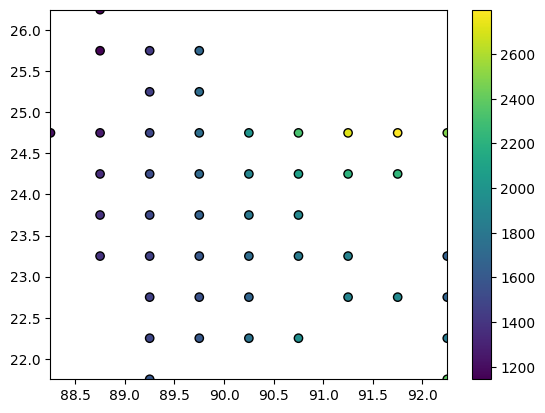In [ ]:
path = "/Users/sapu_a/Desktop/Research/Paper (Bengal Basin)/Dhaka Hydro/New Data/drive-download-20230306T090921Z-001/"

In [ ]:
path = "/Users/sapu_a/Desktop/Research/Paper (Bengal Basin)/Dhaka Hydro/New Data/drive-download-20230306T090921Z-001/"

# Create the figure and axis objects
fig, ax = plt.subplots(1, 1, figsize=(10, 8))

# Plot the shapefile
shapefile.plot(ax=ax, color='gray', edgecolor='black')

# Plot the interpolated rainfall data
contour = ax.contourf(grid_lons, grid_lats, rainfall_interp, cmap='viridis')

# Plot the original data points
scatter = ax.scatter(lons, lats, c=rainfall, edgecolor='k')

plt.colorbar(scatter)

plt.xlabel('Longitude')
plt.ylabel('Latitude')

# Show the plot
plt.show()

/Users/sapu_a/opt/anaconda3/envs/rivtest/lib/python3.8/site-packages/matplotlib/contour.py:1454: UserWarning: Warning: converting a masked element to nan.
self.zmax = float(z.max())
/Users/sapu_a/opt/anaconda3/envs/rivtest/lib/python3.8/site-packages/matplotlib/contour.py:1455: UserWarning: Warning: converting a masked element to nan.
self.zmin = float(z.min())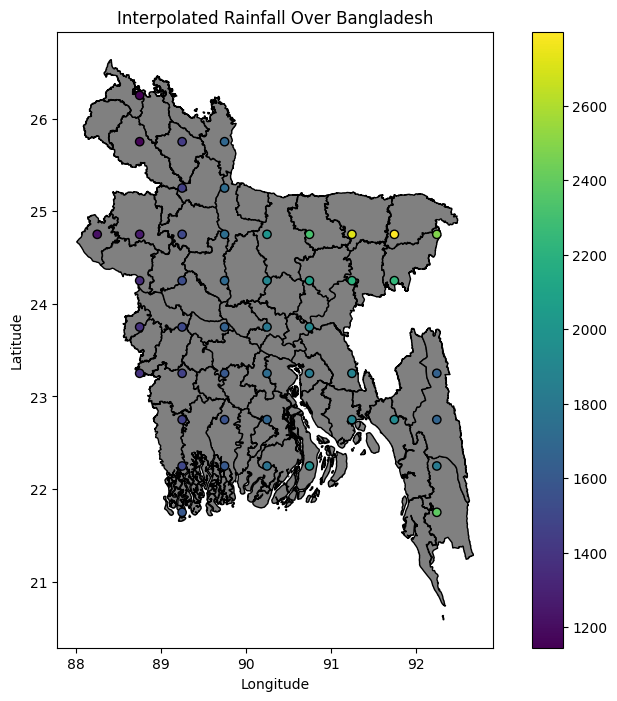In [ ]:
## define following function for basic idw and scipy rbf (Radial Basis Function)

def simple_idw(x, y, z, xi, yi):
dist = distance_matrix(x,y, xi,yi)

# In IDW, weights are 1 / distance
weights = 1.0 / dist

# Make weights sum to one
weights /= weights.sum(axis=0)

# Multiply the weights for each interpolated point by all observed Z-values
zi = np.dot(weights.T, z)
return zi

def distance_matrix(x0, y0, x1, y1):
obs = np.vstack((x0, y0)).T
interp = np.vstack((x1, y1)).T

# Make a distance matrix between pairwise observations
d0 = np.subtract.outer(obs[:,0], interp[:,0])
d1 = np.subtract.outer(obs[:,1], interp[:,1])

return np.hypot(d0, d1)

def scipy_idw(x, y, z, xi, yi):
interp = Rbf(x, y, z, function='linear')
return interp(xi, yi)

def linear_rbf(x, y, z, xi, yi):
dist = distance_matrix(x,y, xi,yi)

# Mutual pariwise distances between observations
internal_dist = distance_matrix(x,y, x,y)

# Now solve for the weights such that mistfit at the observations is minimized
weights = np.linalg.solve(internal_dist, z)

# Multiply the weights for each interpolated point by the distances
zi =  np.dot(dist.T, weights)
return zi

def plot(x, y, z, grid):
plt.figure()
plt.imshow(grid, extent=(x.min(), x.max(), y.min(), y.max()))
plt.scatter(x, y, c=z)
plt.colorbar()
plt.gca().invert_yaxis()

In [ ]:
def main():
# Load the data from a CSV file

# Extract the x, y, and z values
x = df['LON'].values
y = df['LAT'].values
z = df['ANN'].values

# Setup: Generate grid...
nx, ny = 100, 100
xi = np.linspace(x.min(), x.max(), nx)
yi = np.linspace(y.min(), y.max(), ny)
xi, yi = np.meshgrid(xi, yi)
xi, yi = xi.flatten(), yi.flatten()

# Calculate IDW
grid1 = simple_idw(x,y,z,xi,yi)
grid1 = grid1.reshape((ny, nx))

# Calculate scipy's RBF
grid2 = scipy_idw(x,y,z,xi,yi)
grid2 = grid2.reshape((ny, nx))

grid3 = linear_rbf(x,y,z,xi,yi)
grid3 = grid3.reshape((ny, nx))

# Comparisons...
plot(x,y,z,grid1)

plot(x,y,z,grid2)
plt.title("Scipy's Rbf with function=linear")

plot(x,y,z,grid3)

plt.show()

# ... (other functions defined as above)

if __name__ == '__main__':
main()

/var/folders/_g/gg9glpns26lc3md7xd_806k00000gr/T/ipykernel_28770/1083492178.py:8: RuntimeWarning: divide by zero encountered in true_divide
weights = 1.0 / dist
/var/folders/_g/gg9glpns26lc3md7xd_806k00000gr/T/ipykernel_28770/1083492178.py:11: RuntimeWarning: invalid value encountered in true_divide
weights /= weights.sum(axis=0)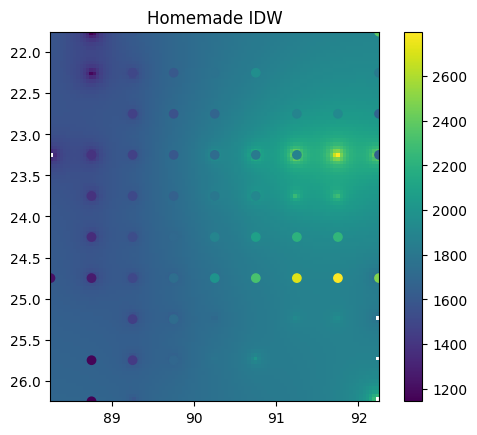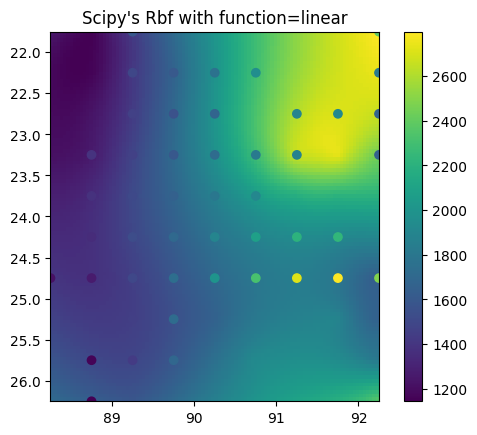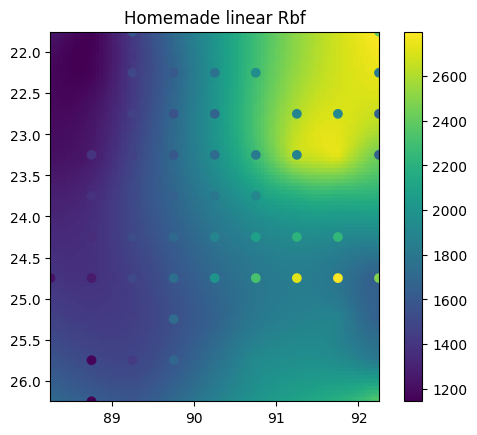In [ ]:
def main():
# Load the data from a CSV file

# Extract the x, y, and z values
x = df['LON'].values
y = df['LAT'].values
z = df['ANN'].values

# Setup: Generate grid...
nx, ny = 500, 500  # increased for denser grid
xi = np.linspace(x.min(), x.max(), nx)
yi = np.linspace(y.min(), y.max(), ny)
xi, yi = np.meshgrid(xi, yi)
xi, yi = xi.flatten(), yi.flatten()

# Calculate IDW
grid1 = simple_idw(x,y,z,xi,yi)
grid1 = grid1.reshape((ny, nx))

# Create a geopandas dataframe of the grid
gdf = gpd.GeoDataFrame(geometry=[Point(x, y) for x, y in zip(xi, yi)], crs=shapefile.crs)

# Create a mask that is True where the grid intersects with the shapefile
mask = np.array([shapefile.geometry.contains(point).any() for point in gdf.geometry]).reshape((ny, nx))

# Apply the mask to the grid

plt.show()

# ... (other functions defined as above)

if __name__ == '__main__':
main()

/var/folders/_g/gg9glpns26lc3md7xd_806k00000gr/T/ipykernel_28770/1083492178.py:8: RuntimeWarning: divide by zero encountered in true_divide
weights = 1.0 / dist
/var/folders/_g/gg9glpns26lc3md7xd_806k00000gr/T/ipykernel_28770/1083492178.py:11: RuntimeWarning: invalid value encountered in true_divide
weights /= weights.sum(axis=0)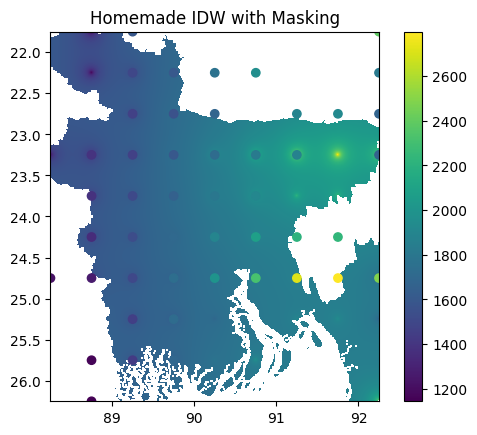In [ ]:
eps = 1e-10  # a small value
xi = np.linspace(x.min(), x.max() + eps, nx)
yi = np.linspace(y.min(), y.max() + eps, ny)

xi_grid, yi_grid = np.meshgrid(xi, yi)

gdf_grid1 = gpd.GeoDataFrame(geometry=[Point(xy) for xy in zip(xi_grid.flatten(), yi_grid.flatten())],
data=grid1.flatten(), columns=['value'])
#gdf_grid2 = gpd.GeoDataFrame(geometry=[Point(xy) for xy in zip(xi_grid.flatten(), yi_grid.flatten())],
#data=grid2.flatten(), columns=['value'])
#gdf_grid3 = gpd.GeoDataFrame(geometry=[Point(xy) for xy in zip(xi_grid.flatten(), yi_grid.flatten())],
#data=grid3.flatten(), columns=['value'])

# Only keep the points that fall within the shapefile
gdf_grid1_within = gdf_grid1[gdf_grid1.geometry.within(shapefile.unary_union)]
#gdf_grid2_within = gdf_grid2[gdf_grid2.geometry.within(shapefile.unary_union)]
#gdf_grid3_within = gdf_grid3[gdf_grid3.geometry.within(shapefile.unary_union)]

# Create full grids of np.nan
zi_grid1_full = np.full((ny, nx), np.nan)
#zi_grid2_full = np.full((ny, nx), np.nan)
#zi_grid3_full = np.full((ny, nx), np.nan)

# Fill in the interpolated values for the points that fall within the shapefile
for gdf, grid in zip([gdf_grid1_within],
[zi_grid1_full]):
for index, row in gdf.iterrows():
point = row['geometry']
value = row['value']
i = np.argmin(np.abs(xi - point.x))
j = np.argmin(np.abs(yi - point.y))
grid[j, i] = value

# Create the figure and axis objects
fig, ax = plt.subplots(1, 1, figsize=(10, 8))

# Plot the shapefile
shapefile.plot(ax=ax, color='gray', edgecolor='black')

# Plot the interpolated values
contour1 = ax.contourf(xi_grid, yi_grid, zi_grid1_full, cmap='viridis')
#contour2 = ax.contourf(xi_grid, yi_grid, zi_grid2_full, cmap='viridis')
#contour3 = ax.contourf(xi_grid, yi_grid, zi_grid3_full, cmap='viridis')

plt.colorbar(contour1, ax=ax)
#plt.colorbar(contour2, ax=ax)
#plt.colorbar(contour3, ax=ax)